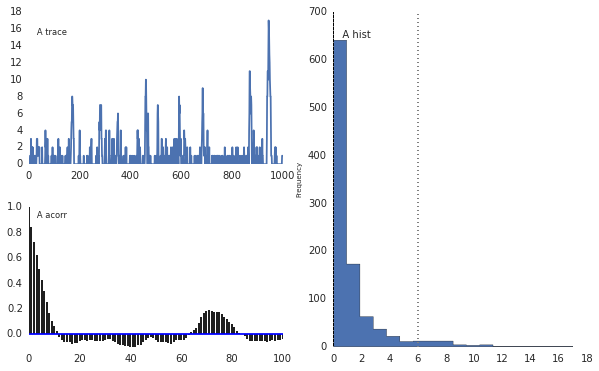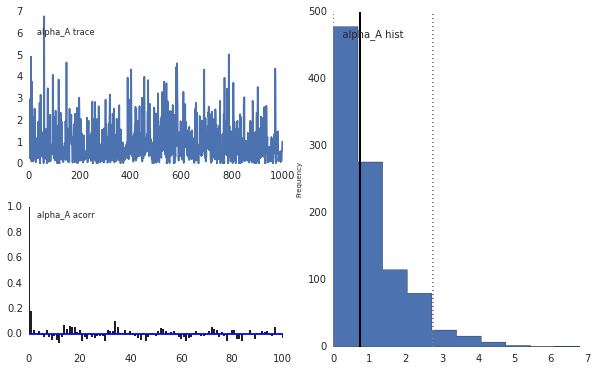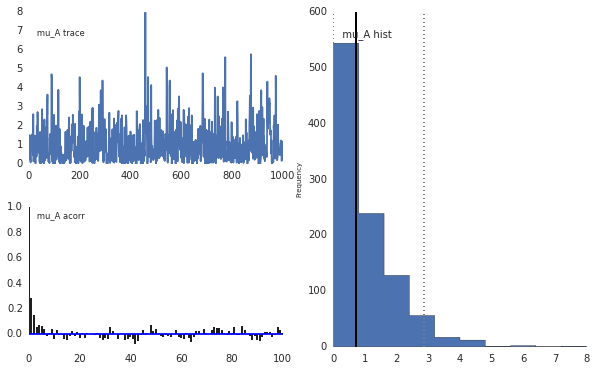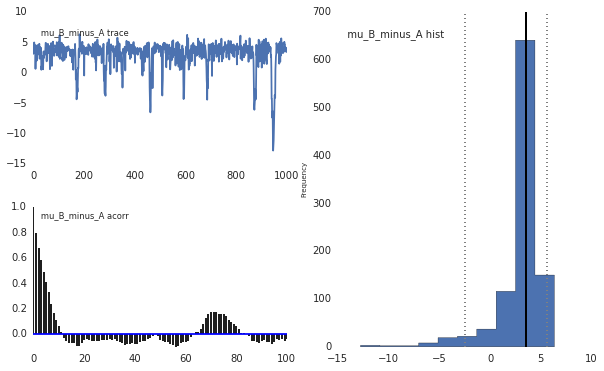In :
!date

Fri Oct 24 11:38:43 PDT 2014


I'm trying to infer models parameters with PyMC. In particular the observed data is modeled as a sum of two different random variables: a negative binomial and a poisson.

In PyMC, an algebraic composition of random variables is described by a "deterministic" object. It is possible to assign the observed data to this deterministic object?

If not possible, we still know that the PDF of the sum is the convolution the PDF of the components. Is there any trick to compute this convolution efficiently?

In :
import numpy as np, pymc as pm, seaborn as sns
%matplotlib inline
np.random.seed(12345) # for reproducibility

In :
def model(values):
# priors for model parameters
mu_A = pm.Exponential('mu_A', beta=1, value=1)
alpha_A = pm.Exponential('alpha_A', beta=1, value=1)
mu_B_minus_A = pm.Uninformative('mu_B_minus_A', value=1)

# latent variable for negative binomial
A = pm.NegativeBinomial('A', mu=mu_A, alpha=alpha_A, value=0)

# observed variable for conditional poisson
B = pm.Poisson('B', mu=mu_B_minus_A+A, value=values, observed=True)

return locals()

In :
n = 10
values = pm.rnegative_binomial(mu=2, alpha=10, size=n) + pm.rpoisson(mu=1, size=n)
m = pm.MCMC(model(values))

m.use_step_method(pm.DiscreteMetropolis, m.A)

In :
m.sample(100000, 50000, 50)
print
pm.Matplot.plot(m)

 [-----------------100%-----------------] 100000 of 100000 complete in 24.3 sec
Plotting A
Plotting alpha_A
Plotting mu_A
Plotting mu_B_minus_AIn :